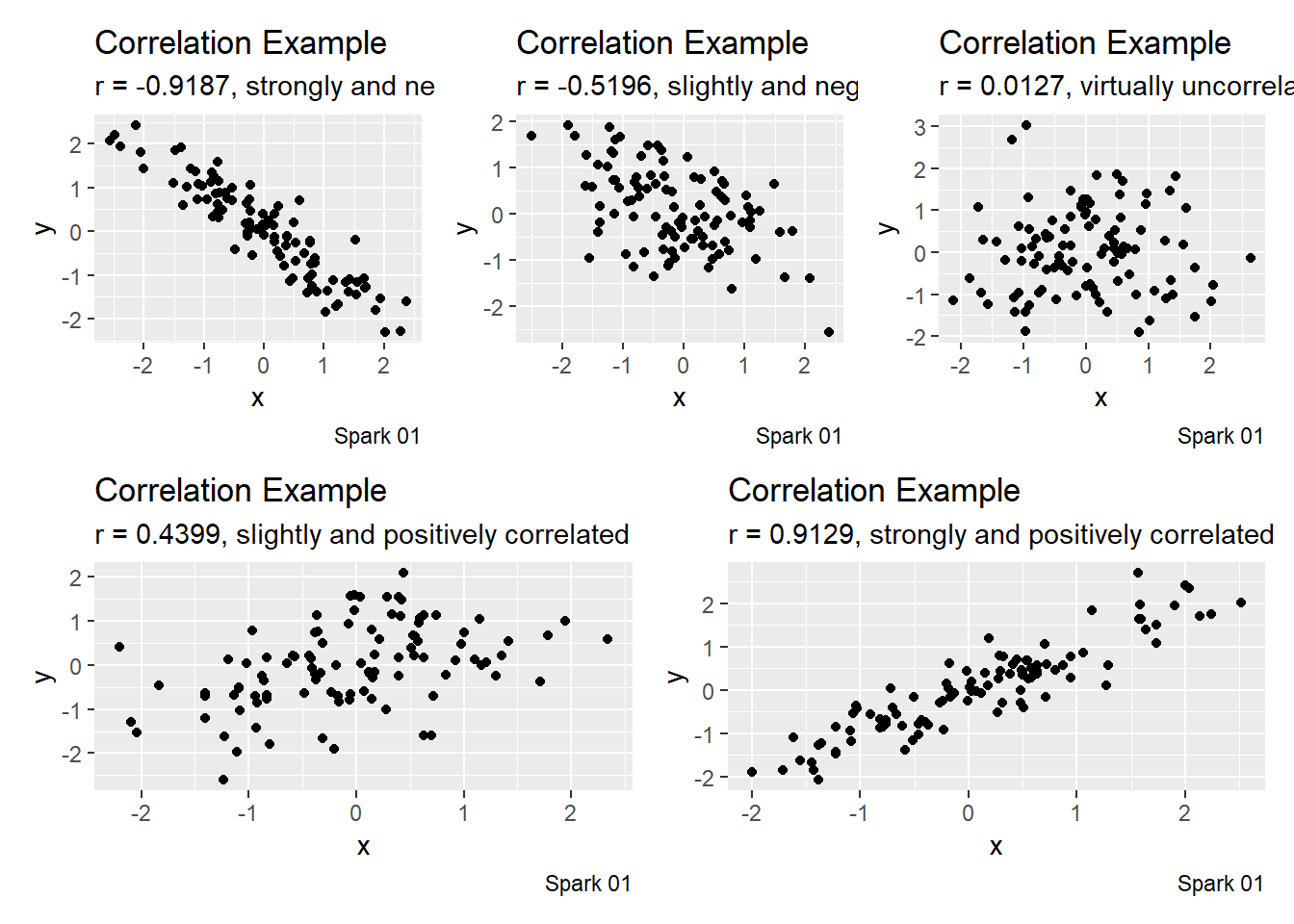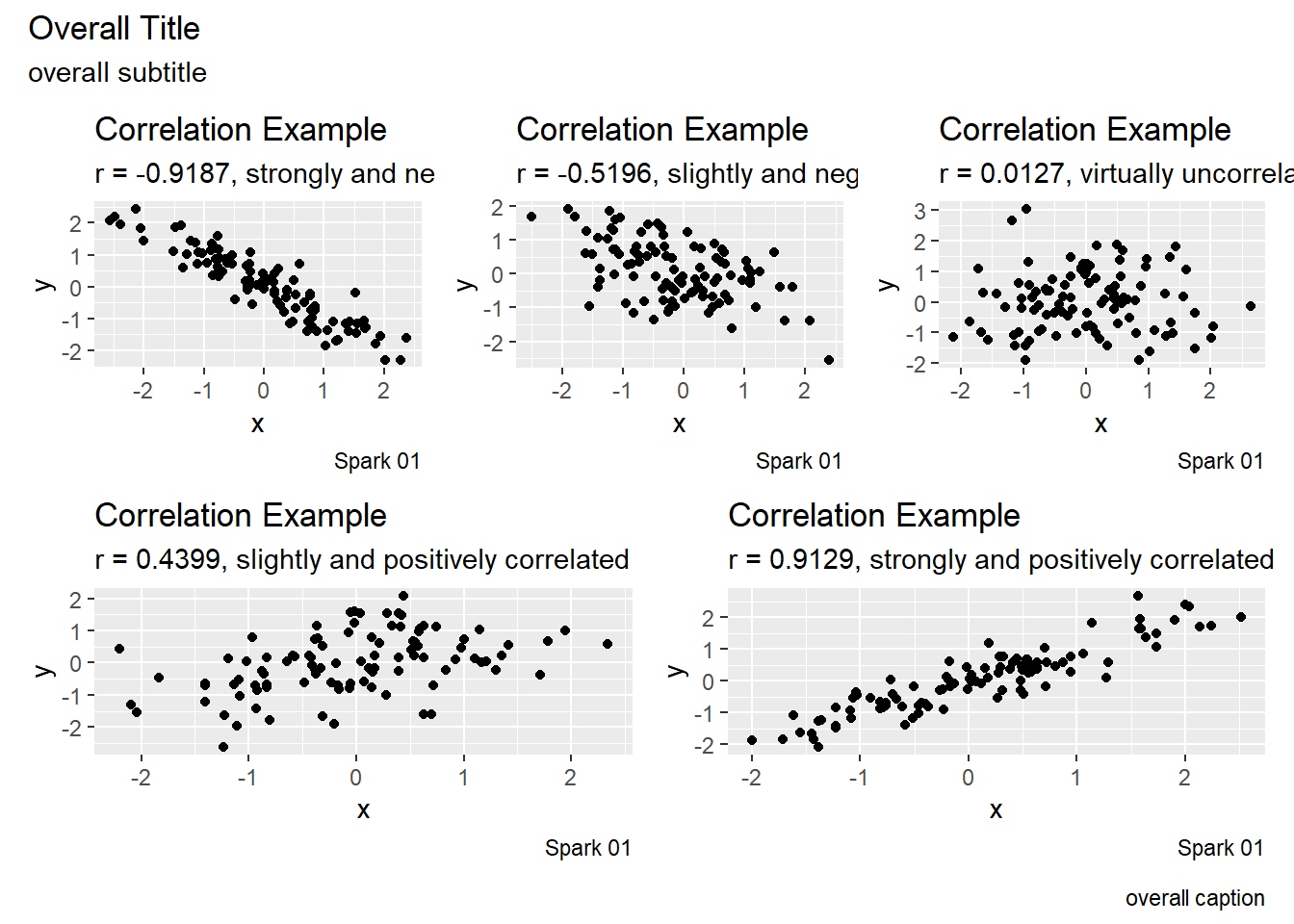# Patchwork

Author

Derek Sollberger

Published

September 27, 2022

In today’s entry, I am simply trying to remember how `patchwork` handles titles of each graph and an overall graph.

``````library("patchwork")
library("tidyverse")``````
``── Attaching packages ─────────────────────────────────────── tidyverse 1.3.1 ──``
``````✔ ggplot2 3.3.6     ✔ purrr   0.3.4
✔ tibble  3.1.8     ✔ dplyr   1.0.9
✔ tidyr   1.2.0     ✔ stringr 1.4.1
✔ readr   2.1.2     ✔ forcats 0.5.1``````
``````── Conflicts ────────────────────────────────────────── tidyverse_conflicts() ──
``````correlatedValues = function(x, r = 0.9){
r2 = r**2
ve = 1-r2
SD = sqrt(ve)
e  = rnorm(length(x), mean=0, sd=SD)
y  = r*x + e
return(y)
}``````

Earlier tonight, I was making these plots for a quick lecture about correlation, so let me just grab some copies.

``````x <- rnorm(100, mean = 0, sd = 1)
y <- correlatedValues(x, r = -0.9)

cor_value <- cor(x,y, use = "pairwise.complete.obs")

df_for_graph <- data.frame(x,y)
p1 <- df_for_graph |>
ggplot(aes(x = x, y = y)) +
geom_point() +
labs(title = "Correlation Example",
subtitle = paste0("r = ", round(cor_value, 4),
", strongly and negatively correlated"),
caption = "Spark 01")``````
``````x <- rnorm(100, mean = 0, sd = 1)
y <- correlatedValues(x, r = -0.5)

cor_value <- cor(x,y, use = "pairwise.complete.obs")

df_for_graph <- data.frame(x,y)
p2 <- df_for_graph |>
ggplot(aes(x = x, y = y)) +
geom_point() +
labs(title = "Correlation Example",
subtitle = paste0("r = ", round(cor_value, 4),
", slightly and negatively correlated"),
caption = "Spark 01")``````
``````x <- rnorm(100, mean = 0, sd = 1)
y <- correlatedValues(x, r = 0)

cor_value <- cor(x,y, use = "pairwise.complete.obs")

df_for_graph <- data.frame(x,y)
p3 <- df_for_graph |>
ggplot(aes(x = x, y = y)) +
geom_point() +
labs(title = "Correlation Example",
subtitle = paste0("r = ", round(cor_value, 4),
", virtually uncorrelated"),
caption = "Spark 01")``````
``````x <- rnorm(100, mean = 0, sd = 1)
y <- correlatedValues(x, r = 0.5)

cor_value <- cor(x,y, use = "pairwise.complete.obs")

df_for_graph <- data.frame(x,y)
p4 <- df_for_graph |>
ggplot(aes(x = x, y = y)) +
geom_point() +
labs(title = "Correlation Example",
subtitle = paste0("r = ", round(cor_value, 4),
", slightly and positively correlated"),
caption = "Spark 01")``````
``````x <- rnorm(100, mean = 0, sd = 1)
y <- correlatedValues(x, r = 0.9)

cor_value <- cor(x,y, use = "pairwise.complete.obs")

df_for_graph <- data.frame(x,y)
p5 <- df_for_graph |>
ggplot(aes(x = x, y = y)) +
geom_point() +
labs(title = "Correlation Example",
subtitle = paste0("r = ", round(cor_value, 4),
", strongly and positively correlated"),
caption = "Spark 01")``````

Now for the `patchwork`

``````#patchwork
(p1 + p2 + p3) / (p4 + p5)``````Now for the annotation.

``````overall_plot <- (p1 + p2 + p3) / (p4 + p5)

overall_plot + plot_annotation(
title = "Overall Title",
subtitle = "overall subtitle",
caption = "overall caption"
)``````Great! Everything is working as planned.